# First Steps With Neural Nets in Keras

The best way to learn an algorithm is to watch it in action. This is why I created the simplest possible neural network in Keras. It's just a single neuron. We will train it on the simplest nonlinear example.

In this post I will explain the basics of neural networks on a visual and conceptual level. I will avoid mathematical calculations and you will find all the code in this post. The important bits of code are shown. The code for the setup and visualizations is collapesed.

The data that we are going to predict is generated by a bilinear function.

$f(x) = \begin{cases} 0 & x \leq 1 \\ 2 \cdot (x-1) & x > 1 \end{cases}$

In :
expand code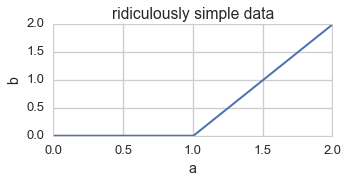This is how a general neuron looks.In our case we will only have one input, the bias and one output. Below you can see how to create one neuron in Keras. The Dense object is the grey circle from the diagram above and the Activation object is the square.

In :
from keras.models import Sequential
from keras.layers.core import Dense, Activation

np.random.seed(0)
model = Sequential()
model.compile(loss='mean_squared_error', optimizer='sgd')

# print initial weigths
weights = model.layers.get_weights()
w0 = weights
w1 = weights
'neural net initialized with weigths w0: {w0:.2f}, w1: {w1:.2f}'.format(**locals())

Out:
'neural net initialized with weigths w0: 0.09, w1: 0.00'

We had to take two choices here. One is the initialization of the weights. We chose them to be randomly drawn from a normal distribution 1. The second choice is the activation function. The chosen ReLu function looks similar to our data.

$ReLu(x) = \begin{cases} 0 & x \leq 0 \\ x & x > 0 \end{cases}$

Training of our neural network is done using back propagation of error. By default the training error in Keras is the mean squared error. The error for our training sample can be written as a big sum depending on the inputs, the weights and the outputs. Now you could change each weight and observe if it reduces the error. If it does, you then change the weight in that direction. The actual algorithm works similar. It just replaces the fiddling with gradient descent. With some time and high school calculus you can derive closed formulas for the updates of the weights.

Now let's train our neuron on the data by calling the fit() method on our model. The collapsed code is storing intermediate values from the training for visualization.

In :
expand code
In :
X_train = np.array(x, ndmin=2).T
Y_train = np.array(y, ndmin=2).T
model.fit(X_train,
Y_train,
nb_epoch=2000,
verbose=0,
callbacks=[history])

# print trained weights
weights = model.layers.get_weights()
w0 = weights
w1 = weights
'neural net weigths after training w0: {w0:.2f}, w1: {w1:.2f}'.format(**locals())

Out:
'neural net weigths after training w0: 1.69, w1: -1.54'
In :
expand code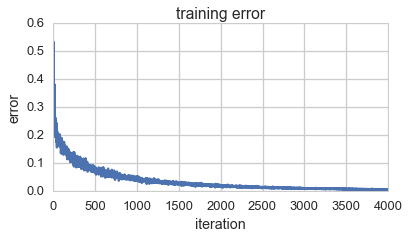I recommend you to use the outputted weights to quickly calculate the neurons predictions for a couple of points.

We can visualize the training by plotting the neurons predictions for each training iteration.

We might be surprised how many iterations it takes to learn such a simple example. Keras is using a learning rate of 0.01 by default. This means in every step it just changes the weights by 1% of the actual change from plain gradient descent. It's a method to prevent overfitting. The net learns slower, but gets better at ignoring noise.

Let's look how the training error evolves with each iteration.

In :
plt.figure(figsize=(6, 3))
plt.plot(history.losses)
plt.ylabel('error')
plt.xlabel('iteration')
plt.title('training error')
plt.show()This might come as a little surprise. While it seemed in the animation like our neuron's predictions where only getting better with every iteration, we see some jitter in the error chart. In some iterations the error actually gets worse. The reason for this is that Keras doesn't do plain gadient descent. For large amounts of data this would be to computanionally expensive.

Instead Keras uses stochastic gradient descent. It randomly selects a subset of our data for each iteration an does a gradient descent on the error on this subset. By default Keras uses 128 data point on each iteration. In a few cases, when the sample would be very skewed, then the optimal weight update for the sample might actually make the predictions worse for the whole data set.

The sample size for stochastic gradient descent is a parameter to the Model.fit() method called batch_size. If we use a larger batch size, we will see a monotonously descereasing error.

In :
expand code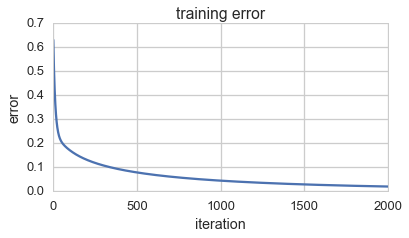How much does our prediction depend on the initial weights? Turns out a lot. This is the same neuron with different initial weigths.

In :
expand code
neural net initialized with weigths w0: -0.02, w1: 0.00
neural net weigths after training w0: -0.02, w1: 0.00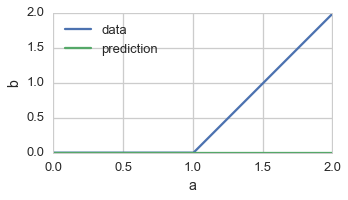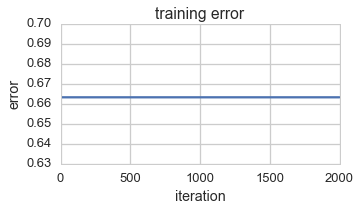The neuron's weights don't get updated during training. This is known as the dying ReLu problem. If the initial weights map all our sample points to values smaller than 0, the ReLu maps everything to 0. Even with small changes in the weights the result is still 0. This means the gradient is 0 and the weights never get updated. MohammedEzz shows you the calculations here. Usually you can mitigate this problem by having a large number of neurons. Some will mostly have non vanishing gradients.

With the understanding of a single neuron, you can move on to more interesting examples in my next post Watch Tiny Neural Nets Learn.

1. Keras uses a normal distribution with mean 0 and standard deviation 0.05.